# Backtracking Sum of Subsets and Knapsack Backtracking Two

• Slides: 36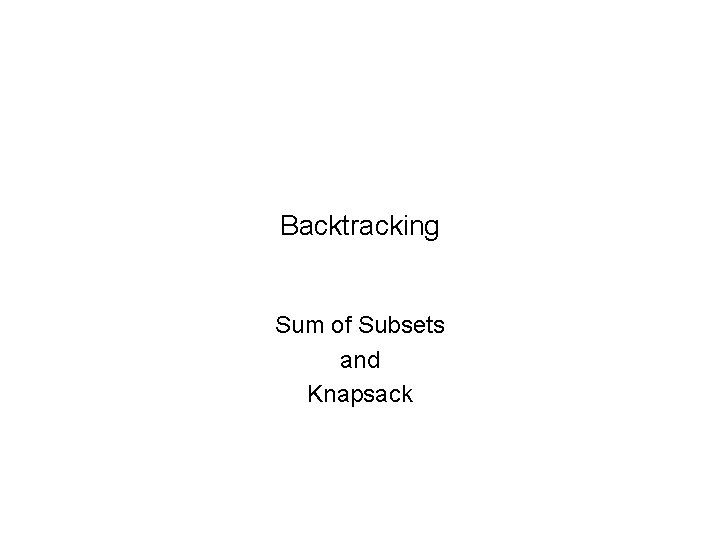Backtracking Sum of Subsets and KnapsackBacktracking • Two versions of backtracking algorithms – Solution needs only to be feasible (satisfy problem’s constraints) • sum of subsets – Solution needs also to be optimal – knapsack 2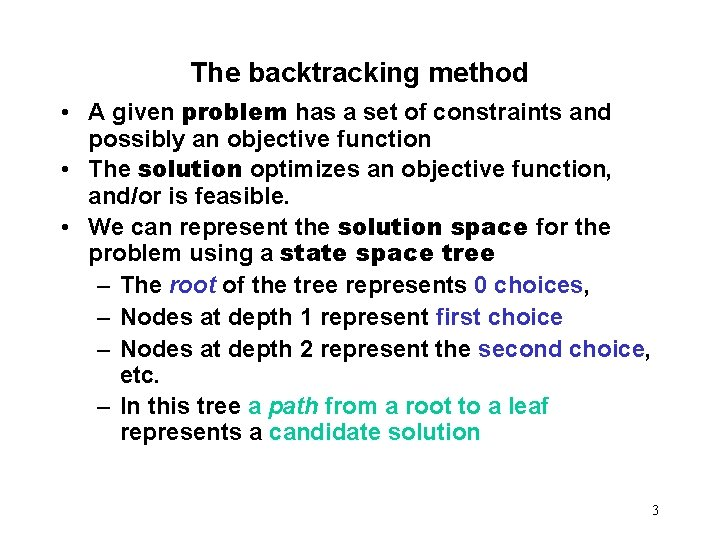The backtracking method • A given problem has a set of constraints and possibly an objective function • The solution optimizes an objective function, and/or is feasible. • We can represent the solution space for the problem using a state space tree – The root of the tree represents 0 choices, – Nodes at depth 1 represent first choice – Nodes at depth 2 represent the second choice, etc. – In this tree a path from a root to a leaf represents a candidate solution 3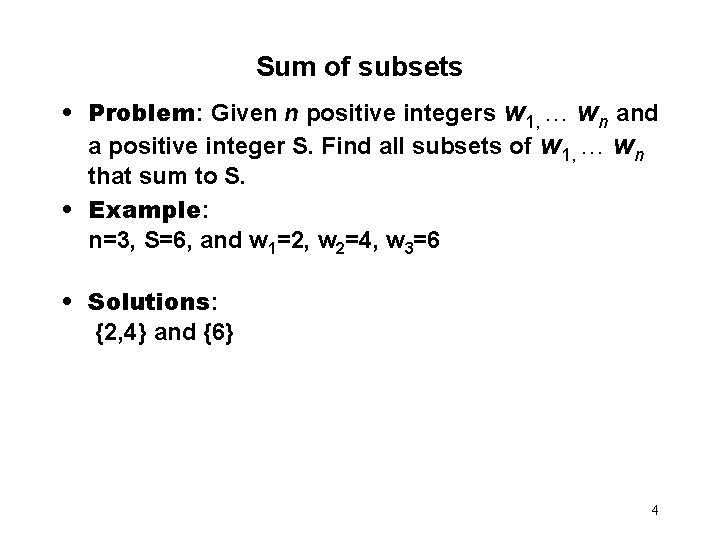Sum of subsets • Problem: Given n positive integers w 1, . . . wn and a positive integer S. Find all subsets of w 1, . . . wn that sum to S. • Example: n=3, S=6, and w 1=2, w 2=4, w 3=6 • Solutions: {2, 4} and {6} 4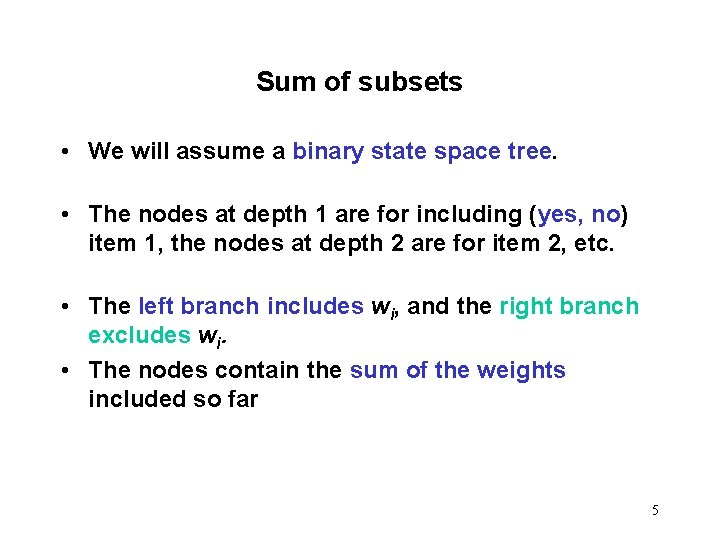Sum of subsets • We will assume a binary state space tree. • The nodes at depth 1 are for including (yes, no) item 1, the nodes at depth 2 are for item 2, etc. • The left branch includes wi, and the right branch excludes wi. • The nodes contain the sum of the weights included so far 5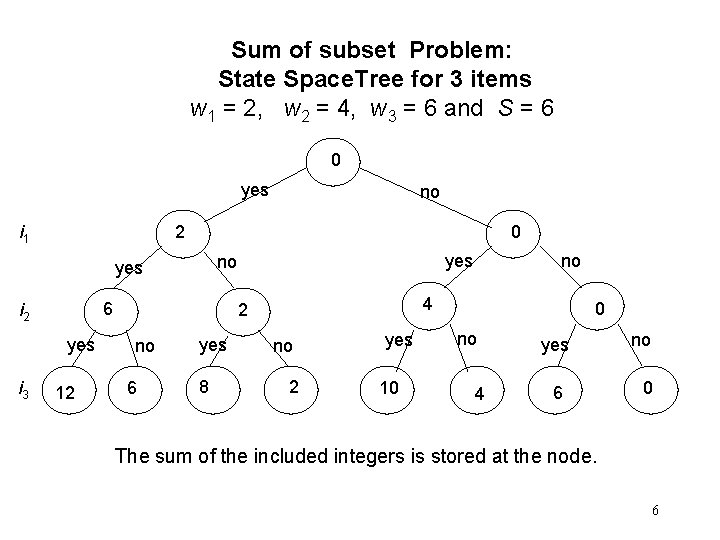Sum of subset Problem: State Space. Tree for 3 items w 1 = 2, w 2 = 4, w 3 = 6 and S = 6 0 yes i 1 2 0 6 i 2 yes 12 yes no yes i 3 no 4 2 no 6 yes 8 no no 2 yes 10 0 no 4 yes 6 no 0 The sum of the included integers is stored at the node. 6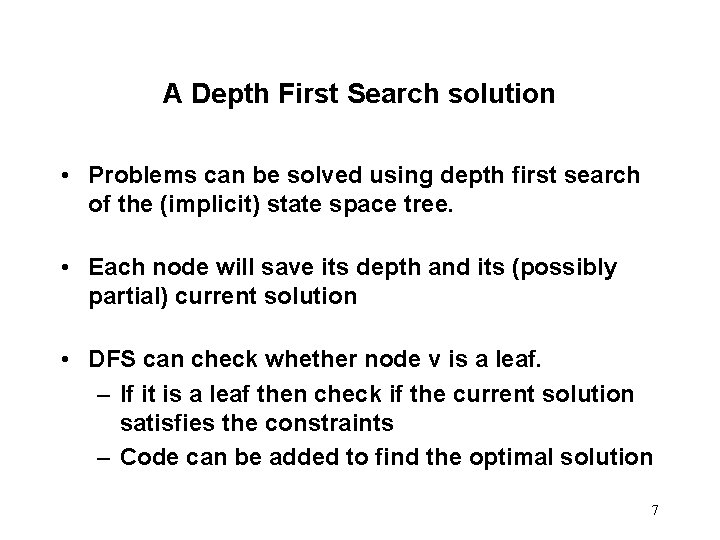A Depth First Search solution • Problems can be solved using depth first search of the (implicit) state space tree. • Each node will save its depth and its (possibly partial) current solution • DFS can check whether node v is a leaf. – If it is a leaf then check if the current solution satisfies the constraints – Code can be added to find the optimal solution 7A DFS solution • Such a DFS algorithm will be very slow. • It does not check for every solution state (node) whether a solution has been reached, or whether a partial solution can lead to a feasible solution • Is there a more efficient solution? 8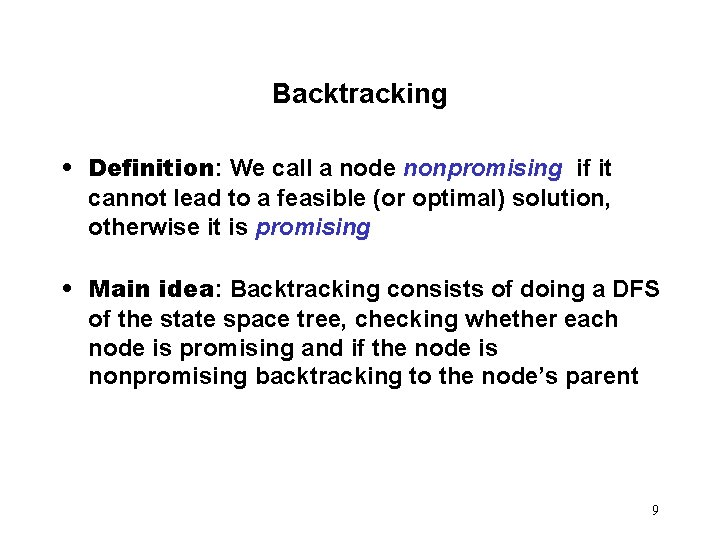Backtracking • Definition: We call a node nonpromising if it cannot lead to a feasible (or optimal) solution, otherwise it is promising • Main idea: Backtracking consists of doing a DFS of the state space tree, checking whether each node is promising and if the node is nonpromising backtracking to the node’s parent 9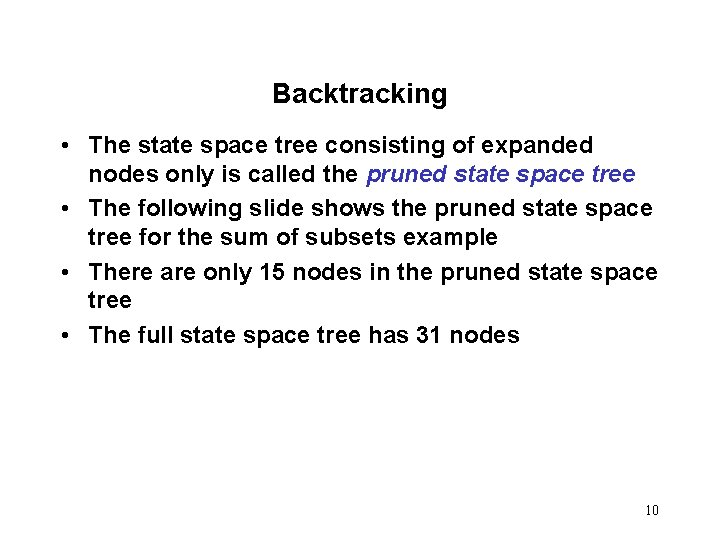Backtracking • The state space tree consisting of expanded nodes only is called the pruned state space tree • The following slide shows the pruned state space tree for the sum of subsets example • There are only 15 nodes in the pruned state space tree • The full state space tree has 31 nodes 10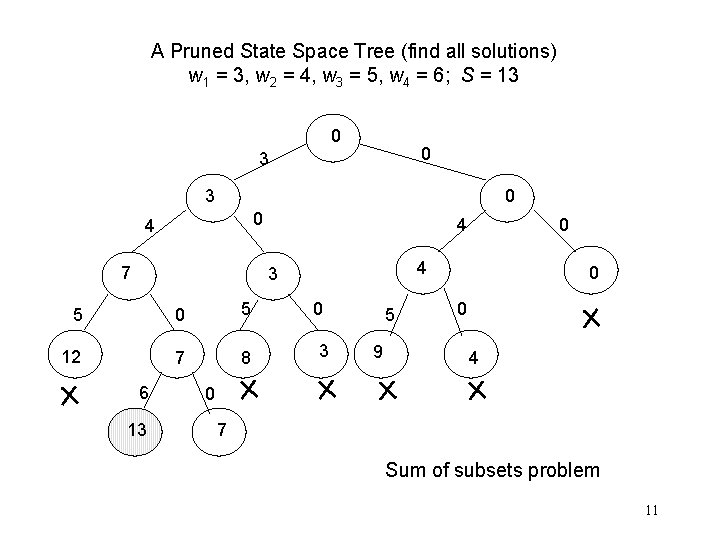A Pruned State Space Tree (find all solutions) w 1 = 3, w 2 = 4, w 3 = 5, w 4 = 6; S = 13 0 0 3 3 0 0 4 7 4 4 3 5 0 5 12 7 8 6 13 0 0 3 5 9 0 0 4 0 7 Sum of subsets problem 11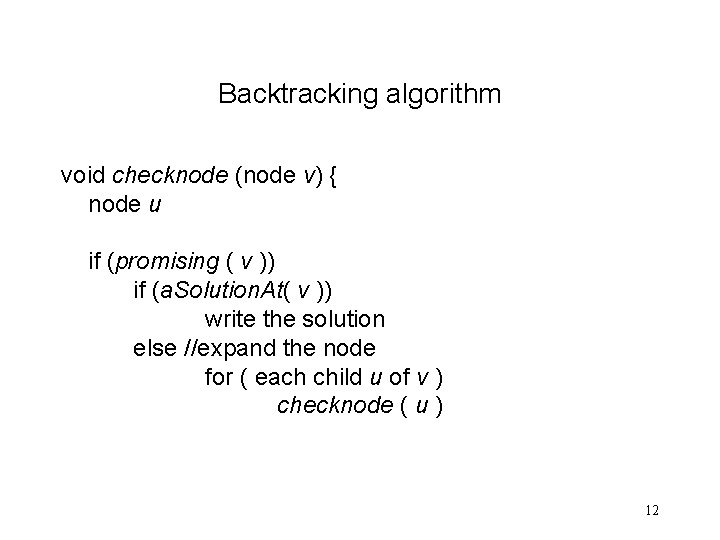Backtracking algorithm void checknode (node v) { node u if (promising ( v )) if (a. Solution. At( v )) write the solution else //expand the node for ( each child u of v ) checknode ( u ) 12Checknode • Checknode uses the functions: – promising(v) which checks that the partial solution represented by v can lead to the required solution – a. Solution. At(v) which checks whether the partial solution represented by node v solves the problem. 13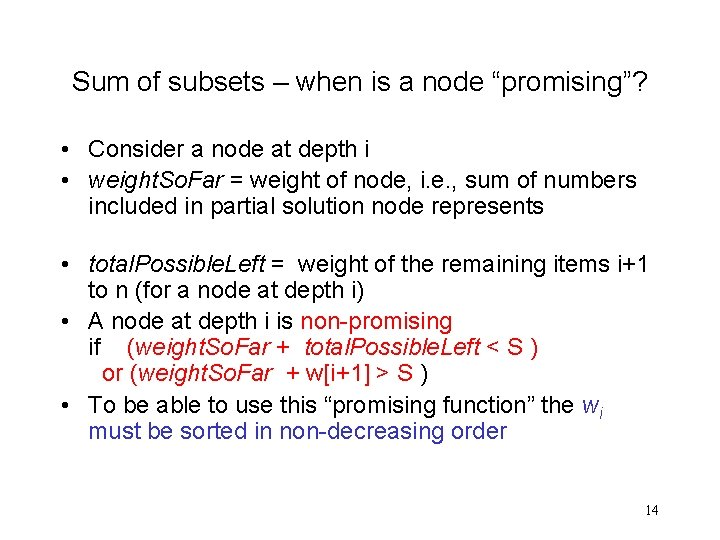Sum of subsets – when is a node “promising”? • Consider a node at depth i • weight. So. Far = weight of node, i. e. , sum of numbers included in partial solution node represents • total. Possible. Left = weight of the remaining items i+1 to n (for a node at depth i) • A node at depth i is non-promising if (weight. So. Far + total. Possible. Left < S ) or (weight. So. Far + w[i+1] > S ) • To be able to use this “promising function” the wi must be sorted in non-decreasing order 14A Pruned State Space Tree w 1 = 3, w 2 = 4, w 3 = 5, w 4 = 6; S = 13 1 0 0 3 2 0 4 3 7 8 5 4 5 7 6 6 13 4 9 8 12 3 5 0 12 11 3 0 10 3 13 9 0 0 4 15 0 0 5 14 4 0 7 7 - backtrack Nodes numbered in “call” order 15sum. Of. Subsets ( i, weight. So. Far, total. Possible. Left ) 1) if (promising ( i )) //may lead to solution 2) then if ( weight. So. Far == S ) 3) then print include[ 1 ] to include[ i ] //found solution 4) else //expand the node when weight. So. Far < S 5) include [ i + 1 ] = "yes” //try including 6) sum. Of. Subsets ( i + 1, weight. So. Far + w[i + 1], total. Possible. Left - w[i + 1] ) 7) include [ i + 1 ] = "no” //try excluding 8) sum. Of. Subsets ( i + 1, weight. So. Far , total. Possible. Left - w[i + 1] ) boolean promising (i ) 1) return ( weight. So. Far + total. Possible. Left S) && ( weight. So. Far == S || weight. So. Far + w[i + 1] S ) Prints all solutions! Initial call sum. Of. Subsets(0, 0, ) 16Backtracking for optimization problems • To deal with optimization we compute: best - value of best solution achieved so far value(v) - the value of the solution at node v – Modify promising(v) • Best is initialized to a value that is equal to a candidate solution or worse than any possible solution. • Best is updated to value(v) if the solution at v is “better” • By “better” we mean: – larger in the case of maximization and – smaller in the case of minimization 17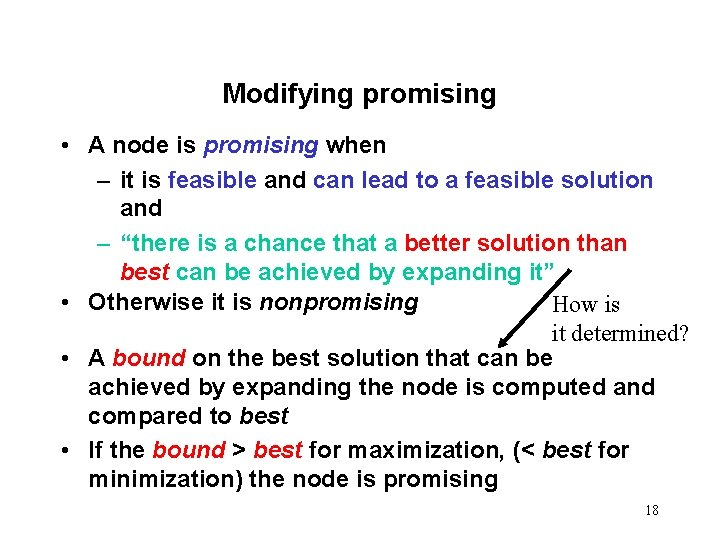Modifying promising • A node is promising when – it is feasible and can lead to a feasible solution and – “there is a chance that a better solution than best can be achieved by expanding it” • Otherwise it is nonpromising How is it determined? • A bound on the best solution that can be achieved by expanding the node is computed and compared to best • If the bound > best for maximization, (< best for minimization) the node is promising 18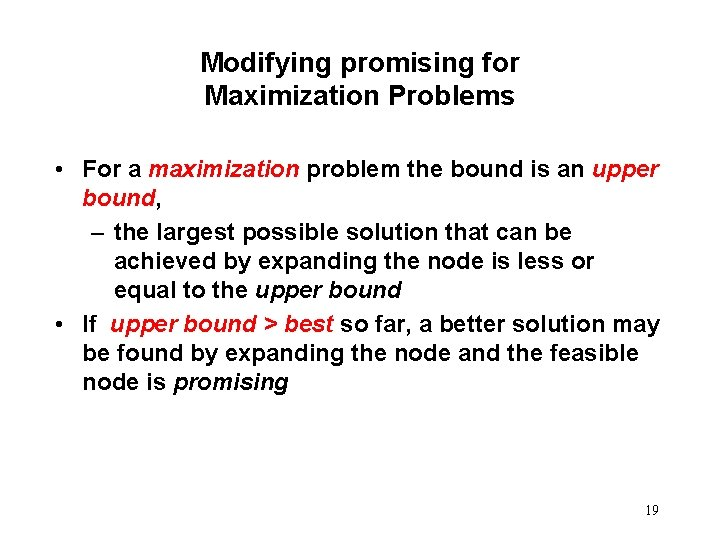Modifying promising for Maximization Problems • For a maximization problem the bound is an upper bound, – the largest possible solution that can be achieved by expanding the node is less or equal to the upper bound • If upper bound > best so far, a better solution may be found by expanding the node and the feasible node is promising 19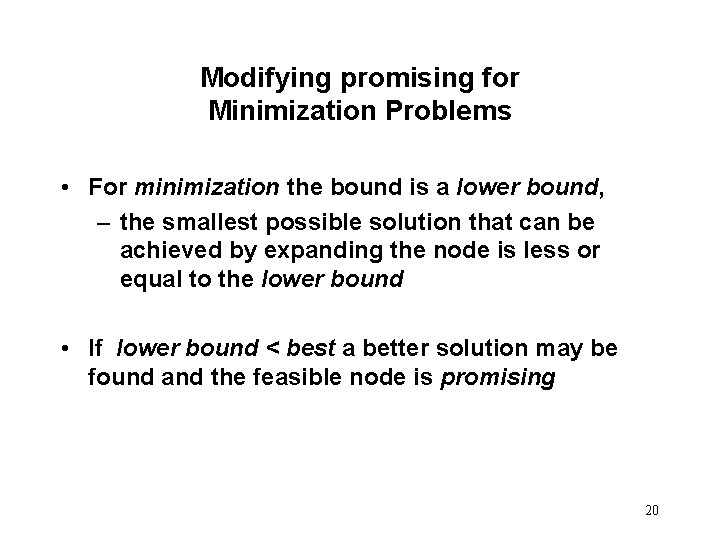Modifying promising for Minimization Problems • For minimization the bound is a lower bound, – the smallest possible solution that can be achieved by expanding the node is less or equal to the lower bound • If lower bound < best a better solution may be found and the feasible node is promising 20Template for backtracking in the case of optimization problems. Procedure checknode (node v ) { node u ; if ( value(v) is better than best ) best = value(v); if (promising (v) ) for (each child u of v) checknode (u ); • best is the best value so far and is initialized to a value that is equal or worse than any possible solution. • value(v) is the value of the solution at the node. } 21Notation for knapsack • We use maxprofit to denote best • profit(v) to denote value(v) 22The state space tree for knapsack • Each node v will include 3 values: – profit (v) = sum of profits of all items included in the knapsack (on a path from root to v) – weight (v)= the sum of the weights of all items included in the knapsack (on a path from root to v) – upper. Bound(v) is greater or equal to the maximum benefit that can be found by expanding the whole subtree of the state space tree with root v. • The nodes are numbered in the order of expansion 23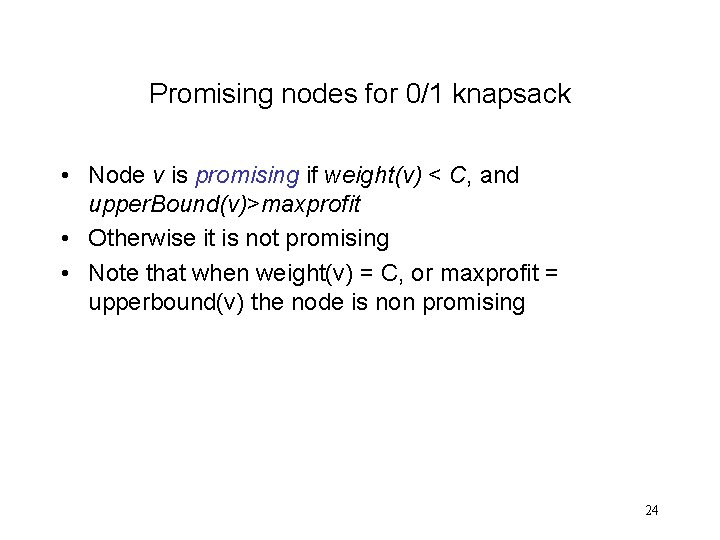Promising nodes for 0/1 knapsack • Node v is promising if weight(v) < C, and upper. Bound(v)>maxprofit • Otherwise it is not promising • Note that when weight(v) = C, or maxprofit = upperbound(v) the node is non promising 24Main idea for upper bound • Theorem: The optimal profit for 0/1 knapsack optimal profit for KWF • Proof: • Clearly the optimal solution to 0/1 knapsack is a possible solution to KWF. So the optimal profit of KWF is greater or equal to that of 0/1 knapsack • Main idea: KWF can be used for computing the upper bounds 25Computing the upper bound for 0/1 knapsack • Given node v at depth i. • Upper. Bound(v) = KWF 2(i+1, weight(v), profit(v), w, p, C, n) • KWF 2 requires that the items be ordered by non increasing pi / wi, so if we arrange the items in this order before applying the backtracking algorithm, KWF 2 will pick the remaining items in the required order. 26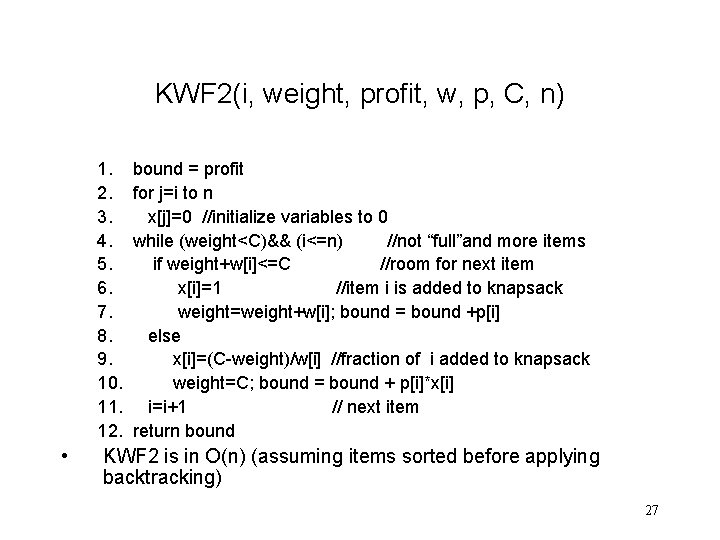KWF 2(i, weight, profit, w, p, C, n) 1. 2. 3. 4. 5. 6. 7. 8. 9. 10. 11. 12. • bound = profit for j=i to n x[j]=0 //initialize variables to 0 while (weight<C)&& (i<=n) //not “full”and more items if weight+w[i]<=C //room for next item x[i]=1 //item i is added to knapsack weight=weight+w[i]; bound = bound +p[i] else x[i]=(C-weight)/w[i] //fraction of i added to knapsack weight=C; bound = bound + p[i]*x[i] i=i+1 // next item return bound KWF 2 is in O(n) (assuming items sorted before applying backtracking) 27C++ version • The arrays w, p, include and bestset have size n+1. • Location 0 is not used • include contains the current solution • bestset the best solution so far 28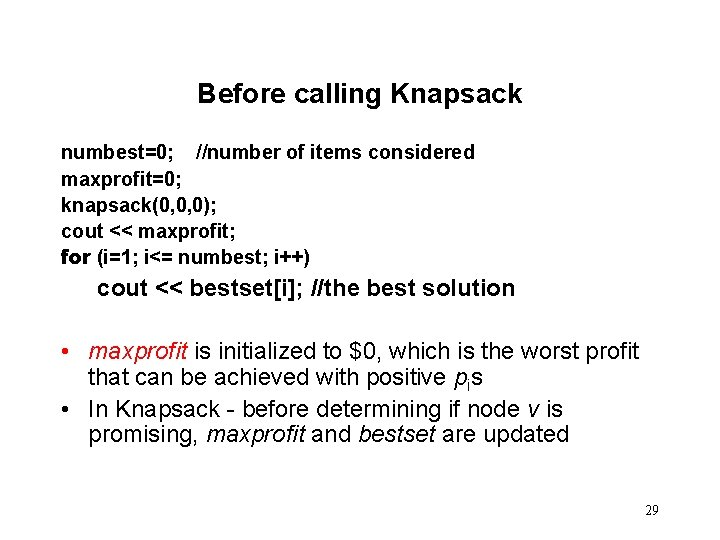Before calling Knapsack numbest=0; //number of items considered maxprofit=0; knapsack(0, 0, 0); cout << maxprofit; for (i=1; i<= numbest; i++) cout << bestset[i]; //the best solution • maxprofit is initialized to \$0, which is the worst profit that can be achieved with positive pis • In Knapsack - before determining if node v is promising, maxprofit and bestset are updated 29knapsack(i, profit, weight) if ( weight <= C && profit > maxprofit) // save better solution maxprofit=profit //save new profit numbest= i; bestset = include//save solution if promising(i) include [i + 1] = “ yes” knapsack(i+1, profit+p[i+1], weight+ w[i+1]) include[i+1] = “no” knapsack(i+1, profit, weight) 30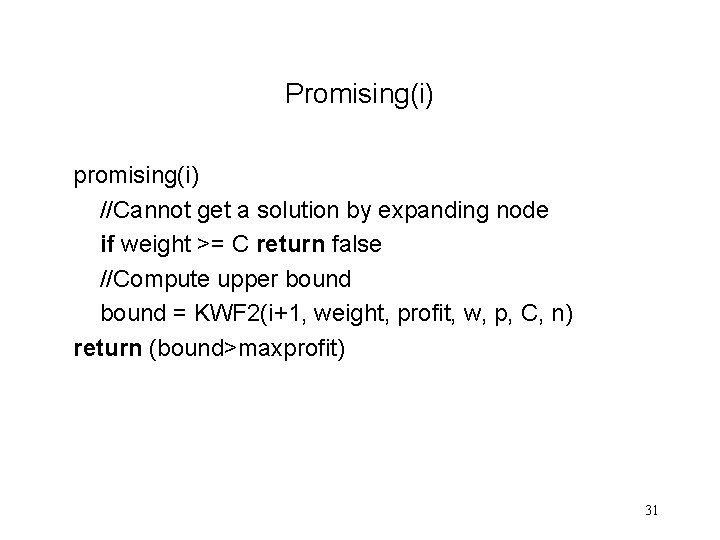Promising(i) promising(i) //Cannot get a solution by expanding node if weight >= C return false //Compute upper bound = KWF 2(i+1, weight, profit, w, p, C, n) return (bound>maxprofit) 31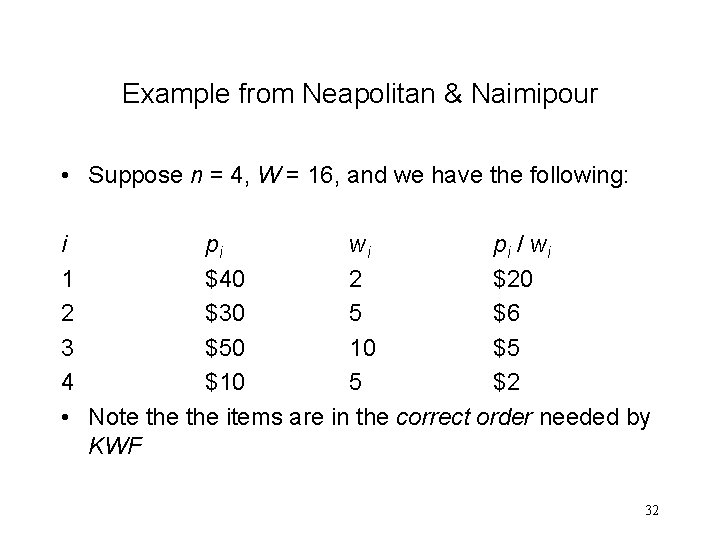Example from Neapolitan & Naimipour • Suppose n = 4, W = 16, and we have the following: i pi wi pi / wi 1 \$40 2 \$20 2 \$30 5 \$6 3 \$50 10 \$5 4 \$10 5 \$2 • Note the items are in the correct order needed by KWF 32The calculation for node 1 maxprofit = \$0 (n = 4, C = 16 ) Node 1 a) profit = \$ 0 weight = 0 b) bound = profit + p 1 + p 2 + (C - 7 ) * p 3 / w 3 = \$0 + \$40 + \$30 + (16 -7) X \$50/10 =\$115 c) 1 is promising because its weight =0 < C = 16 and its bound \$115 > 0 the value of maxprofit. 33The calculation for node 2 Item 1 with profit \$40 and weight 2 is included maxprofit = \$40 a) profit = \$40 weight = 2 b) bound = profit + p 2 + (C - 7) X p 3 / w 3 = \$40 + \$30 + (16 -7) X \$50/10 =\$115 c) 2 is promising because its weight =2 < C = 16 and its bound \$115 > \$40 the value of maxprofit. 34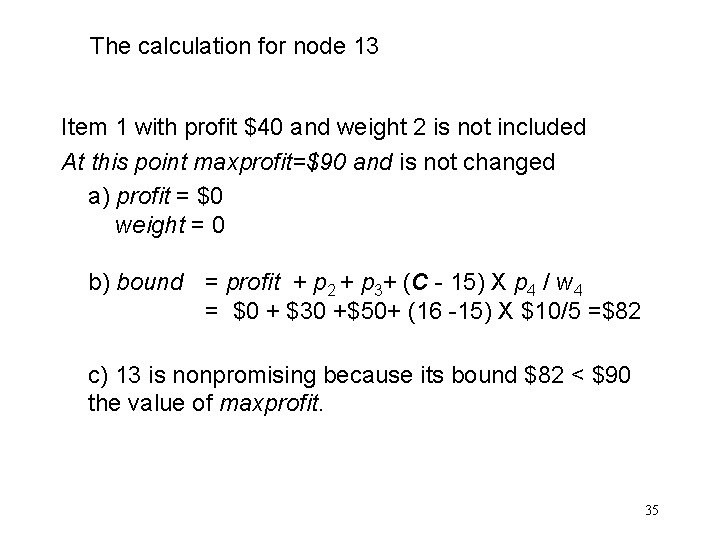The calculation for node 13 Item 1 with profit \$40 and weight 2 is not included At this point maxprofit=\$90 and is not changed a) profit = \$0 weight = 0 b) bound = profit + p 2 + p 3+ (C - 15) X p 4 / w 4 = \$0 + \$30 +\$50+ (16 -15) X \$10/5 =\$82 c) 13 is nonpromising because its bound \$82 < \$90 the value of maxprofit. 35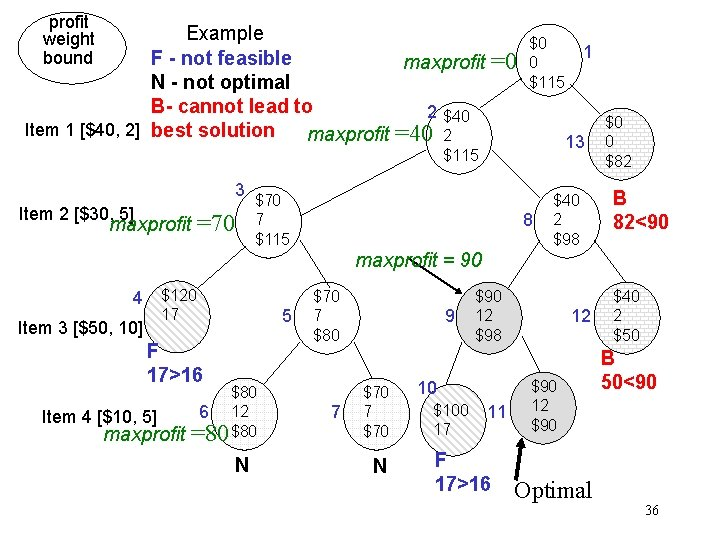profit weight bound Example F - not feasible maxprofit N - not optimal B- cannot lead to 2 \$40 Item 1 [\$40, 2] best solution maxprofit =40 2 =0 \$0 0 \$115 13 \$115 3 Item 2 [\$30, 5] =70 maxprofit 1 \$70 7 \$115 8 \$40 2 \$98 \$0 0 \$82 B 82<90 maxprofit = 90 4 \$120 17 Item 3 [\$50, 10] 5 F 17>16 Item 4 [\$10, 5] maxprofit \$80 6 12 =80 \$80 N \$70 7 \$80 7 9 \$70 7 \$70 N \$90 12 \$98 10 \$100 17 11 F 17>16 12 \$90 Optimal \$40 2 \$50 B 50<90 36